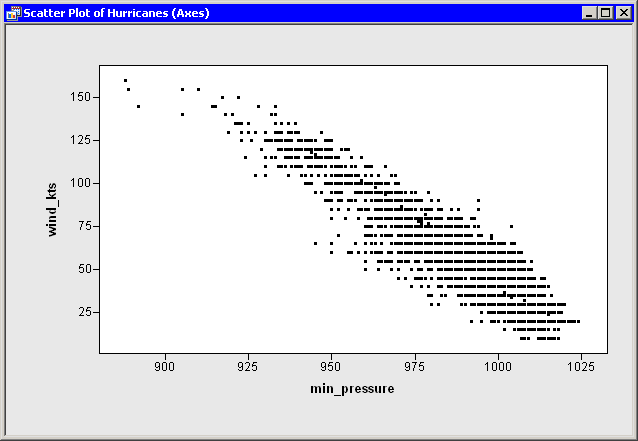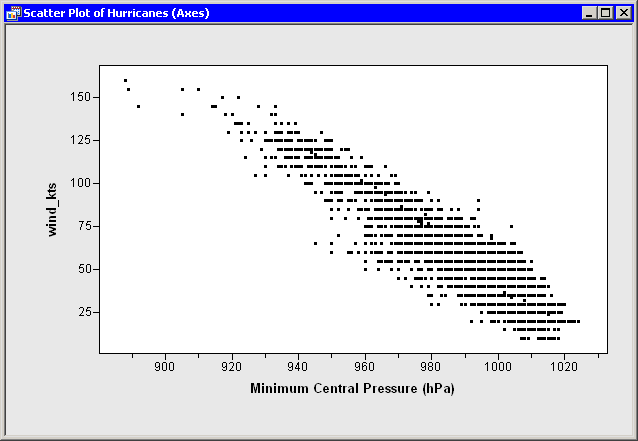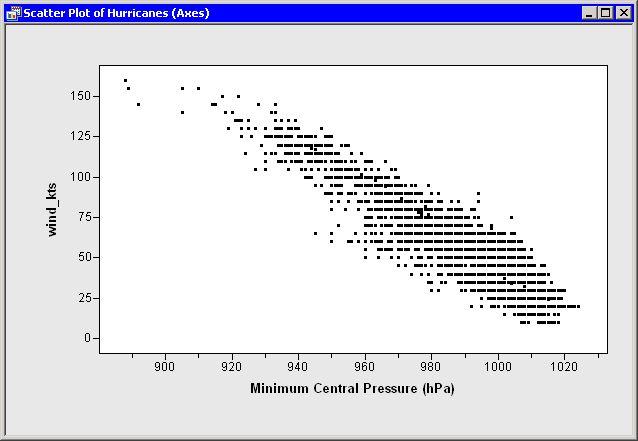Adjusting Axes and Locations of Ticks

# Change Axes Properties and Tick Locations

First, open the Hurricanes data set and create a scatter plot. Type or copy the following statements into the program window, and select ProgramRun from the main menu.

```
declare DataObject dobj;
dobj = DataObject.CreateFromFile("Hurricanes");

declare ScatterPlot plot;
plot = ScatterPlot.Create( dobj, "min_pressure", "wind_kts" );
```

The plot in Figure 9.1 appears. SAS/IML Studio tries to make appropriate choices for the location of ticks and for the minimum and maximum of the axis view range. However, sometimes you might want to change characteristics of the axes.

Figure 9.1 Default AxesThere are several methods in the Plot class that can be used to change the way that axes are displayed. These methods all begin with the prefix SetAxis. For example:

• The SetAxisLabel method changes the axis label.

• The SetAxisTickUnit method changes the increment between tick marks.

• The SetAxisTickAnchor method shifts the location of tick marks along an axis. (The argument to SetTickAnchor must be a number between the minimum and maximum values of the axis tick range.)

• The SetAxisMinorTicks method adds minor tick marks (that is, unlabeled tick marks between major ticks).

These and other methods that affect axes are documented in the SAS/IML Studio online Help.

Suppose you want to change the horizontal axis in the following ways:

• Label the axis with the variable’s label instead of the variable’s name.

• Change the size of the increment between successive ticks from 25 to 20 so that the ticks marks are located at 900, 920, ..., 1020.

• Add one minor tick mark between each major tick.

To make these changes, add the following statements at the bottom of the program window, and select ProgramRun from the main menu. Figure 9.2 shows the new label, minor ticks, and tick placement for the horizontal axis.

```
plot.SetAxisLabel ( XAXIS, AXISLABEL_VARLABEL );
plot.SetAxisTickUnit( XAXIS, 20 );
plot.SetAxisTickAnchor( XAXIS, 900 );
plot.SetAxisMinorTicks( XAXIS, 1 );
```

Figure 9.2 New Horizontal AxisBy default, the axis view range is determined by the minimum and maximum values of the axis variable. (More precisely, it is determined by the minimum and maximum values that are included in the plots.) At times you might want to override that default. A common reason for doing this is to include a reference value (for example, zero) in the plot, even though there are no observations with that value.

As an example of changing the axis view range, suppose you want the vertical axis (the wind_kts axis) to show zero wind speed as in Figure 9.3. To change the ticks on the horizontal axis, add the following statement at the bottom of the program window, and select ProgramRun from the main menu.

```
plot.SetAxisViewRange( YAXIS, 0, 160 );
```

Figure 9.3 Vertical Axis Range That Includes ZeroPrevious Page | Next Page | Top of Page